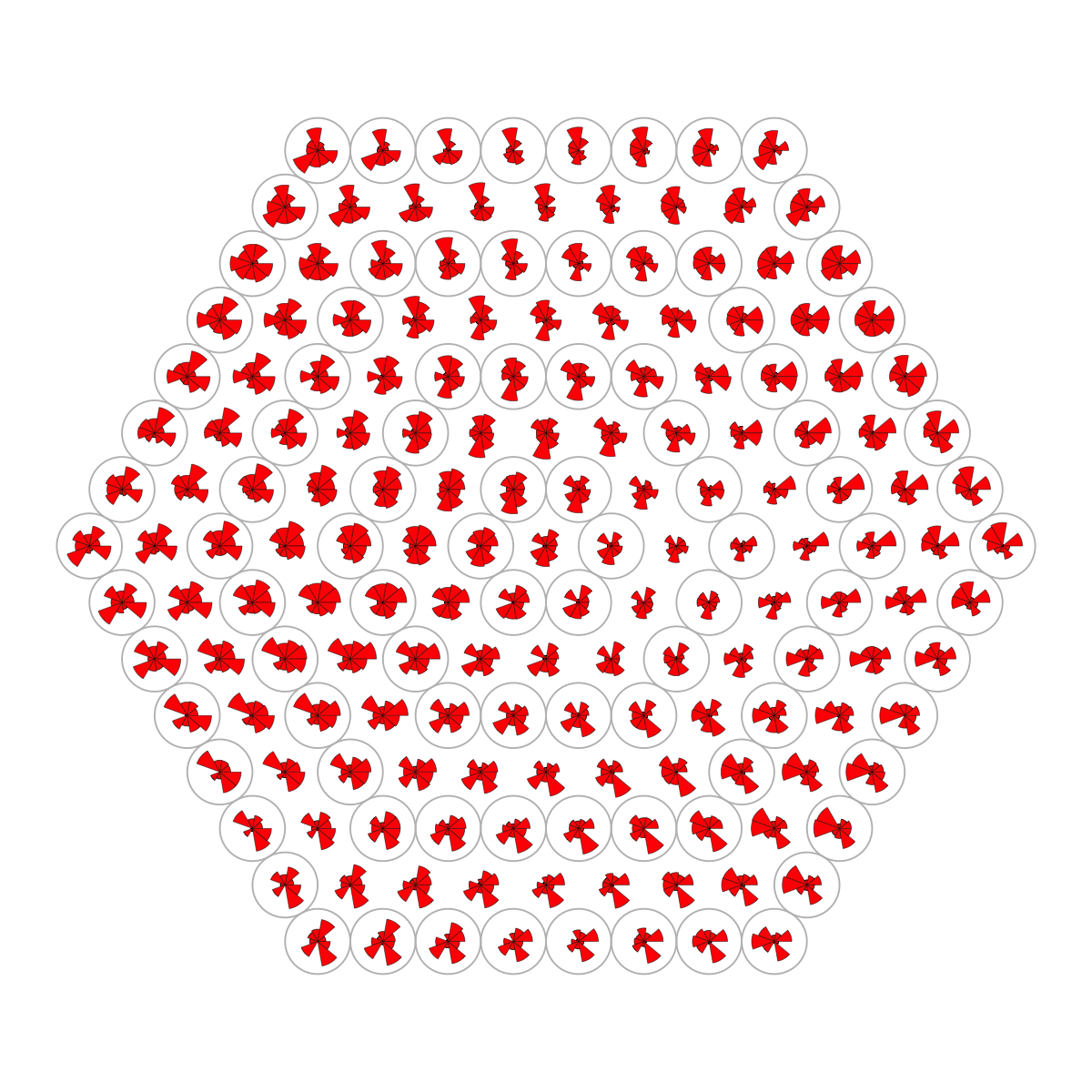## Function to visualise codebook matrix or input patterns within a supra-hexagonal grid

### Description

`visHexPattern` is supposed to codebook matrix or input patterns within a supra-hexagonal grid.

### Usage

```visHexPattern(sObj, plotType = c("lines", "bars", "radars"), pattern = NULL, height = 7,
margin = rep(0.1, 4), colormap = c("customized", "bwr", "jet", "gbr", "wyr",
"br", "yr", "rainbow", "wb"), customized.color = "red", alterntive.color = c("transparent",
"gray"), zeropattern.color = "gray", legend = TRUE, legend.cex = 0.8, legend.label = NULL,
newpage = TRUE)```

### Arguments

sObj
an object of class "sMap" or "sTopol" or "sInit"
plotType
the plot type, can be "lines" for line/point graph, "bars" for bar graph, "radars" for radar graph
pattern
By default, it sets to "NULL" for the codebook matrix. It is intended for the user-input patterns, i.e., a matrix with the dimension of nHex x nPattern, where nHex is the number of hexagons and nPattern is the number of elements for each pattern
height
a numeric value specifying the height of device
margin
margins as units of length 4 or 1
colormap
short name for the predifined colormap, and "customized" for custom input (see the next 'customized.color'). The predifined colormap can be one of "jet" (jet colormap), "bwr" (blue-white-red colormap), "gbr" (green-black-red colormap), "wyr" (white-yellow-red colormap), "br" (black-red colormap), "yr" (yellow-red colormap), "wb" (white-black colormap), and "rainbow" (rainbow colormap, that is, red-yellow-green-cyan-blue-magenta). Alternatively, any hyphen-separated HTML color names, e.g. "blue-black-yellow", "royalblue-white-sandybrown", "darkgreen-white-darkviolet". A list of standard color names can be found in http://html-color-codes.info/color-names
customized.color
the customized color for pattern visualisation
alterntive.color
the alterntive color used to indicate the hexagon layout
zeropattern.color
the color for zero horizental line
legend
logical to indicate whether to add the legend
legend.cex
a numerical value giving the amount by which legend text should be magnified relative to the default (i.e., 1)
legend.label
a vector specifying the legend label. By default, it is NULL for using column names of the codebook matrix (or the matrix given by the parameter 'pattern')
newpage
logical to indicate whether to open a new page. By default, it sets to true for opening a new page

invisible

### Note

The "plotType" includes:

• "lines": line plot. If multple colors are given, the points are also plotted. When the pattern involves both positive and negative values, zero horizental line is also shown
• "bars": bar plot. When the pattern involves both positive and negative values, the zero horizental line is in the middle of the hexagon; otherwise at the top of the hexagon for all negative values, and at the bottom for all positive values
• "radars": radar plot. Each radar diagram represents one pattern, wherein each element value is proportional to the distance from the center. Note, it starts on the right and wind counterclockwise around the circle

### Examples

```# 1) generate data with an iid matrix of 1000 x 9
data <- cbind(matrix(rnorm(1000*3,mean=0,sd=1), nrow=1000, ncol=3),
matrix(rnorm(1000*3,mean=0.5,sd=1), nrow=1000, ncol=3),
matrix(rnorm(1000*3,mean=-0.5,sd=1), nrow=1000, ncol=3))
colnames(data) <- c("S1","S1","S1","S2","S2","S2","S3","S3","S3")

# 2) sMap resulted from using by default setup
sMap <- sPipeline(data=data)

Start at 2018-01-18 16:56:32

First, define topology of a map grid (2018-01-18 16:56:32)...
Second, initialise the codebook matrix (169 X 9) using 'linear' initialisation, given a topology and input data (2018-01-18 16:56:32)...
Third, get training at the rough stage (2018-01-18 16:56:32)...
1 out of 2 (2018-01-18 16:56:32)
updated (2018-01-18 16:56:32)
2 out of 2 (2018-01-18 16:56:32)
updated (2018-01-18 16:56:32)
Fourth, get training at the finetune stage (2018-01-18 16:56:32)...
1 out of 7 (2018-01-18 16:56:32)
updated (2018-01-18 16:56:32)
2 out of 7 (2018-01-18 16:56:32)
updated (2018-01-18 16:56:32)
3 out of 7 (2018-01-18 16:56:32)
updated (2018-01-18 16:56:32)
4 out of 7 (2018-01-18 16:56:32)
updated (2018-01-18 16:56:32)
5 out of 7 (2018-01-18 16:56:32)
updated (2018-01-18 16:56:32)
6 out of 7 (2018-01-18 16:56:32)
updated (2018-01-18 16:56:32)
7 out of 7 (2018-01-18 16:56:32)
updated (2018-01-18 16:56:32)
Next, identify the best-matching hexagon/rectangle for the input data (2018-01-18 16:56:32)...
Finally, append the response data (hits and mqe) into the sMap object (2018-01-18 16:56:32)...

Below are the summaries of the training results:
dimension of input data: 1000x9
xy-dimension of map grid: xdim=15, ydim=15, r=8
grid lattice: hexa
grid shape: suprahex
dimension of grid coord: 169x2
initialisation method: linear
dimension of codebook matrix: 169x9
mean quantization error: 4.22315848559725

Below are the details of trainology:
training algorithm: batch
alpha type: invert
training neighborhood kernel: gaussian
trainlength (x input data length): 2 at rough stage; 7 at finetune stage
radius (at rough stage): from 4 to 1
radius (at finetune stage): from 1 to 1

End at 2018-01-18 16:56:32
Runtime in total is: 0 secs

# 3) plot codebook patterns using different types
# 3a) line plot
visHexPattern(sMap, plotType="lines")
# 3b) bar plot
visHexPattern(sMap, plotType="bars")
# 4) plot user-input patterns using different types
# 4a) generate pattern data with two different groups "S" and "T"
nHex <- sMap\$nHexpattern <- cbind(matrix(runif(nHex*3,min=0,max=1), nrow=nHex, ncol=3),
matrix(runif(nHex*3,min=1,max=2), nrow=nHex, ncol=3))
colnames(pattern) <- c("S1","S2","S3","T1","T2","T3")
# 4b) for line plot
visHexPattern(sMap, plotType="lines", pattern=pattern,
customized.color="red", zeropattern.color="gray")
# 4c) for bar plot
visHexPattern(sMap, plotType="bars", pattern=pattern,
customized.color=rep(c("red","green"),each=3))
visHexPattern(sMap, plotType="bars", pattern=pattern,
customized.color=rep(c("red","green"),each=3), legend.label=c("S","T"))
customized.color=rep(c("red","green"),each=3))
customized.color=rep(c("red","green"),each=3), legend.label=c("S","T"))
```

## Source code

`visHexPattern.r`

## Source man

`visHexPattern.Rd` `visHexPattern.pdf`

`sPipeline`, `visColormap`